# The derivative of a function to find the derivative of the function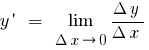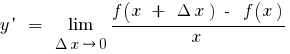Definition: the Derivative of the function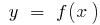at the pointis called the limit of the ratio of the increment of the function at pointto the increment of the argument, when the increment of the argument tends to zero (to denoteor)

The operation of finding a derivative is called differentiation

## The concept of the growth of argument and function

The growth argument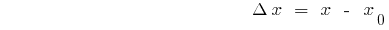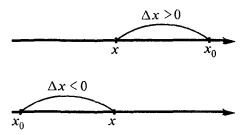The increment function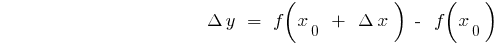## Derivatives of elementary functions

Derivatives of elementary functions are found using the table: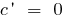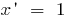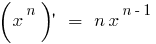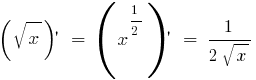All the derivatives of elementary functions can be found here!

## Compiled function. How to find compiled function

Derivative of sum (difference) of two functions, each of which has a derivative equal to the sum (difference) of the derivatives of these functions: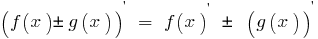Derivative works of two functions, each of which has a derivative equal to the sum of the products of each function into derivative the second function: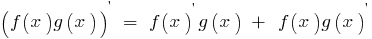Derivative shares shares of the two functions f(x) and g(x), each of which has derivative g(x)≠0, is given byThe constant factor can be moved from the sign of the derivative: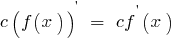Formulas called rules of differentiation.

## The geometric meaning of derivative

The tangent to the curve at a given pointis called the limiting position of the secant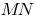, when the pointis approaching along a curve to the point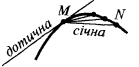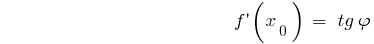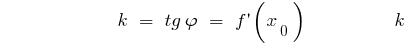— the slope of the tangent

The equation of the tangent to the graph of the function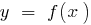at the point with Alsou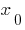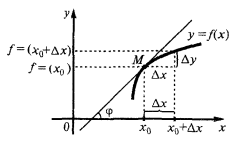The value of the derivative at the pointis equal to the angular coefficient of the tangent to the graph of the function at the point with Alsouand equal to the tangent of the angle of inclination of this tangent to the axis## Physical meaning of the derivative

The derivative describes the rate of change of the function when you change the argument— the dependence distance traveled from the time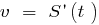speed rectilinear motionacceleration rectilinear motion

Tags:
Chapter:
Versions in other languages:
Share with friends: